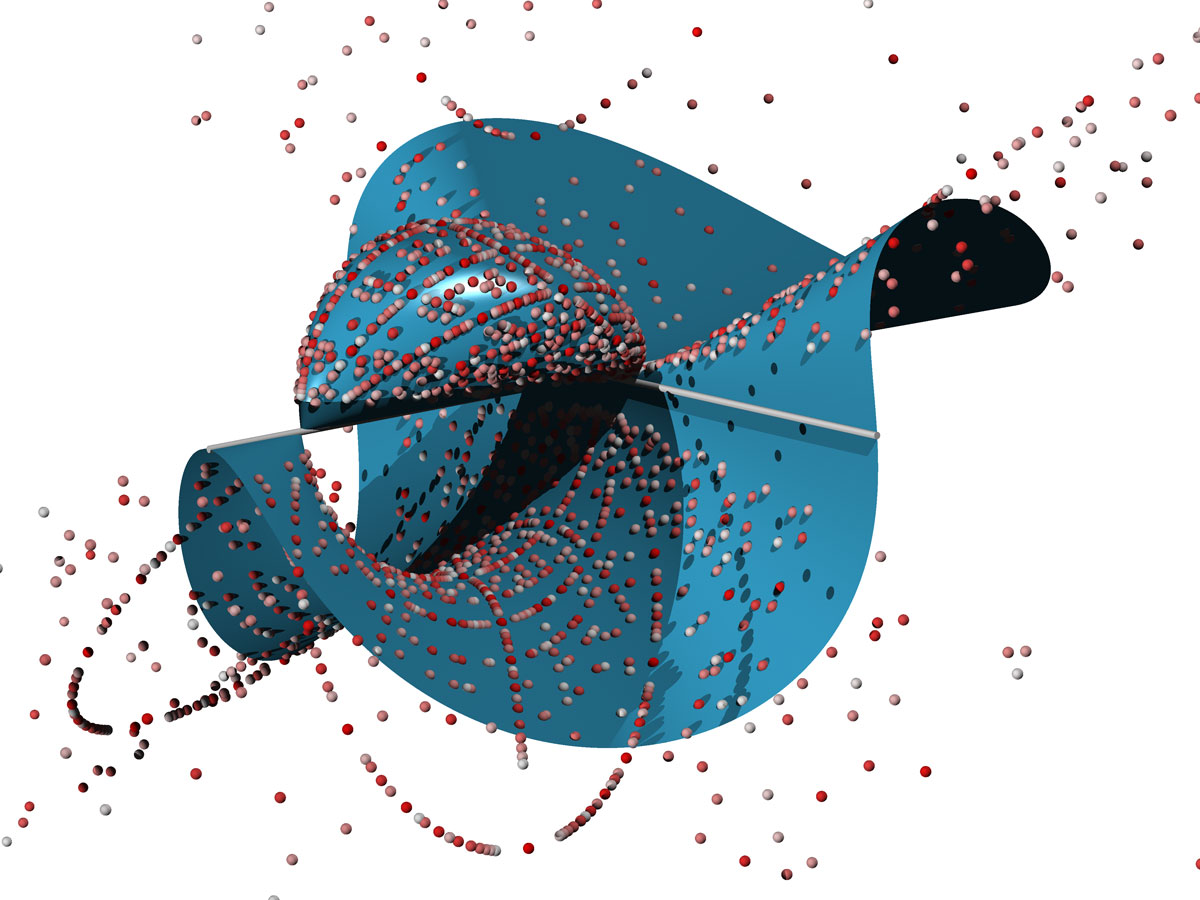August 17, 2020

# When is necessary sufficient?

## Researchers provide the key to an algorithm for finding integer solutions to certain polynomial equations.The cubic surface x³+xy+yz²=0 passes the two tests and has numerous integer solutions (red dots), but the conjecture remains open for cubic equations in general. © Ulrich Derenthal / Leibniz University Hannover

In 1900, Hilbert famously challenged researchers to design an algorithm that could say whether or not a polynomial equation with integer coefficients has an integer solution. We now know that this, his so-called tenth problem, is in general impossible. However, a new theorem by Institute of Science and Technology Austria (IST Austria) Professor Tim Browning and his coauthors, Professor Pierre Le Boudec at the University of Basel and Professor Will Sawin at Columbia University, has provided the key to creating such an algorithm for nearly all polynomials whose number of variables is greater than their degree. In so doing, they almost entirely resolve a central conjecture in number theory. Their results have been announced on arXiv.org and submitted for publication.

Polynomials with integer coefficients and the integer solutions thereof—known as Diophantine equations—have been studied at least as far back as the Babylonians, and continue to fascinate us today. One example involves solutions to the equation x3+y3+z3=k, for integer values of k. Mathematicians spent decades looking for integer solutions for k less than 100; only last year were the final few k<100 solved. Though finding specific integer solutions to given polynomial equations is an exciting area, the very existence of solutions is also an important question.

If a polynomial equation does have integer solutions, two conditions must hold, one having to do with solutions that are real numbers, the other with divisibility by integers. A central conjecture in number theory, proposed in 2001, suggested that if the number of variables (call this number n) of a polynomial is greater than the degree (call this number d), then passing these two tests almost always means there exists an integer solution. “In a way, the conjecture describes when necessary local properties—the two tests—are sufficient to make global statements about solvability,” explains Browning.

“I first heard about this problem just after I had finished my PhD, and it’s been on my mind ever since,” recalls Browning. Real progress came only 16 years later, when Browning and Le Boudec had the idea to look at the size of a typical solution. “The idea was that if the expected size of a solution is too large, then it was probably the case that there isn’t a solution at all,” Browning explains. Using this, the two were able to prove a large part of the conjecture but they still could not address infinitely many polynomials that passed the two tests. Unwilling to settle for this, the two started collaborating with their third coauthor, Will Sawin, who helped to build the two tests much more efficiently into the argument.

In the end, Browning and his coauthors proved the conjecture to be true in all cases except when n=4 and d=3, a family known as cubic surfaces. The team’s theorem can be combined with an algorithm that decides whether a polynomial passes the two tests mentioned above. Together, they give strong evidence that there is an algorithm deciding whether or not a typical polynomial with n>d has integer solutions.

Browning and his group continue to study cubic surfaces, among other Diophantine equations. Together, they seek to understand what polynomials pass the two tests above, but fail to have integer solutions. It is conjectured that a third test—the Brauer-Manin obstruction—is sufficient to explain the remaining cases; Browning and his group are working to resolve this conjecture. “While not achieving Hilbert’s impossible dream, it is possibly as close as we can get for integer solutions,” Browning adds.

### Publication

T. Browning, P. Le Boudec, and W. Sawin. 2020. The Hasse principle for random Fano hypersurfaces. arXiv:2006.02356.